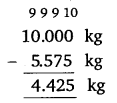# Class 6 Maths NCERT Solutions for Chapter 8 Decimals Ex – 8.6

## Decimals

Question 1.
Subtract:

(a) ₹ 18.25 from ₹ 20.75
(b) ₹ 202.54 m from 250 m
(c) ₹ 5.36 from ₹ 8.40
(d) 2.051 km from 5.206 km
(e) 0.314 kg from 2.107 kg

Solution: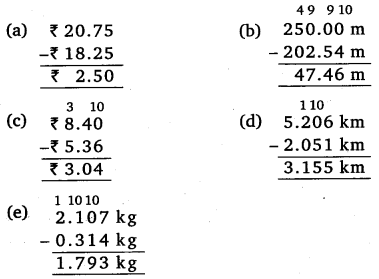Question 2.
Find the value of:

(a) 9.756 – 6.28
(b) 21.05 – 15.27
(c) 18.5 – 6.79
(d) 11.6 – 9.

Solution:Question 3.
Raju bought a book for ₹ 35.65. He gave ₹ 50 to the shopkeeper. How much money did he get back from the shopkeeper?

Solution:
Cost of the book = ₹ 35.65
Money given to the shopkeeper by Raju = ₹ 50
Money he got back = ₹ 50 – ₹ 35.65 = ₹ 14.35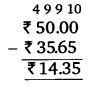Question 4.
Rani had ₹ 18.50. She bought one ice-cream for ₹ 11.75. How much money does she have now?

Solution:
Money with Rani = ₹ 18.50
Money spent on ice-cream=₹ 11.75
Money left with Rani = ₹ 18.50 – ₹ 11.75 = ₹ 6.75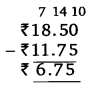Question 5.
Tina had 20 m 5 cm long cloth. She cuts 4 m 50 cm length of cloth from this for making a curtain. How much cloth is left with her?

Solution:
Total length of cloth = 20 m 5 cm = 20.05 m
Length cut out for curtain = 4 m 50 cm = 4.50 m
Cloth left over = 20.05 m – 4.50 m = 15.55 m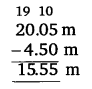Question 6.
Namita travels 20 km 50 m every day. Out of this she travels 10 km 200 m by bus and the rest by auto. How much distance does she travel by auto?

Solution:
Total distance travelled by Namita
20 km 50 m = 20.050 km
Distance travelled by bus = 10 km 200 m = 10.200 km
∴ Distance travelled by auto = 20.050 km – 10.200 km
= 9.850 km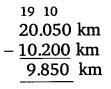Question 7.
Aakash bought vegetables weighing 10 kg. Out of this, 3 kg 500 g is onions, 2 kg 75 g is tomatoes and the rest is potatoes. What is the weight of the potatoes?

Solution:
Total weight of vegetables bought = 10 kg
Weight of onions = 3 kg 500 g = 3.500 kg
Weight of tomato= 2 kg 75 g = 2.075 kg
∴ Total weight of these vegetables =3.500 kg + 2.075 kg = 5.575 kg
As per question
Weight of potatoes = 10 kg – 5.575 kg = 4.425 kg